# Join operation in DBMS

When we have to combine one or more column filters in the WHERE clause we can use them by using AND or OR operators. The AND operator will perform logical AND on the filter conditions specified and displays the result, where as OR will perform logical OR operations.

Suppose we want to retrieve the student records who are in class ‘DESIGN_01’ and have address as Troy. The query for the same with AND condition is as below:

```SELECT STUDENT _ID, STUDENT_NAME, ADDRESS, CLASS_ID
FROM STUDENT s
WHERE s.CLASS_ID = ‘DESIGN_01’

The same query with OR condition would be

```SELECT STUDENT _ID, STUDENT_NAME, ADDRESS, CLASS_ID
FROM STUDENT s
WHERE s.CLASS_ID = ‘DESIGN_01’

What is the difference between the two queries above? The first query retrieves the students who are in class DESIGN_01 and coming from Troy. Whereas second query retrieves the students who are either in class DESIGN_01 or coming from Troy. The result set of both the queries are different as shown below: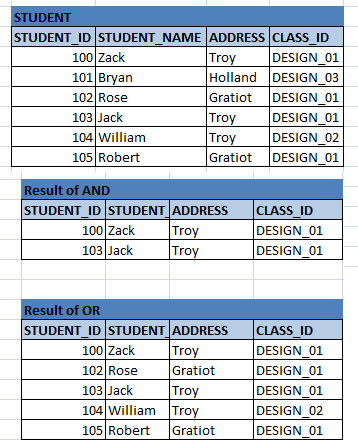In addition to AND and OR operators, we can join one or more tables based on special conditions. Like we saw in relational algebra, same is in SQL – inner join, left outer join, right outer join and full join. It works on the table similar to relational algebra.

## Inner Join

This is the most common type of join and is similar to AND operation explained above. It combines the results of one or more tables and displays the results when all the filter conditions are met. There are two ways to represent the same.

```SELECT STUDENT _ID, std_mark
FROM STUDENT s, MARKS m
WHERE s.STUENT_ID = m.STUDENT_ID;```
```SELECT STUDENT _ID, std_mark
FROM STUDENT s INNER JOIN MARKS m
ON s.STUENT_ID = m.STUDENT_ID;```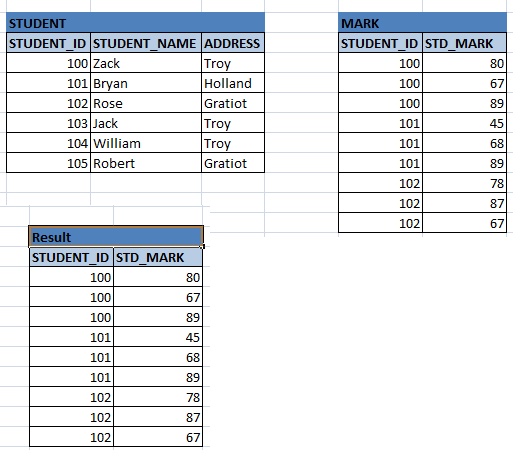Here it combines the two tables STUDENT and MARKS. It displays the result only if there is matching students in both the tables. In the above query we can even specify simply ‘JOIN’ instead of INNER JOIN to represent the same.

## Left Outer Join

This join retrieves all the records from the table which is on the LHS of ‘LEFT OUTER JOIN’ clause and only the matching records from RHS.

Below example of left outer join on DEPT and EMPLOYEE table combines the matching combination of DEPT_ID = 10 with values. But DEPT_ID = 30 does not have any employees yet. Hence it displays NULL for those employees. Thus this outer join makes more meaningful to combining two relations than a cartesian product.

```SELECT d.DEPT_ID,
d.DEPT_NAME,
e.EMP_ID,
e.ENAME,
e.DEPT_ID
FROM DEPT d, EMP e
WHERE d.DEPT_ID = e.DEPT_ID (+);```
```SELECT d.DEPT_ID,
d.DEPT_NAME,
e.EMP_ID,
e.ENAME,
e.DEPT_ID
FROM DEPT d LEFT OUTER JOIN EMP e
ON d.DEPT_ID = e.DEPT_ID;```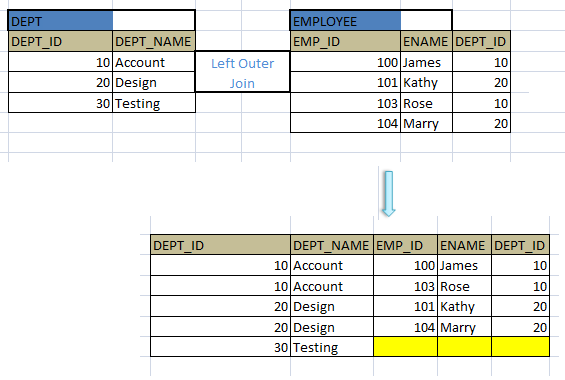## Right Outer Join

This join is opposite of left outer join. It retrieves all the records from the table which is on the RHS of ‘RIGHT OUTER JOIN’ clause and only the matching records from LHS.

Same example as above when re-written with Right outer join is as below:

```SELECT d.DEPT_ID,
d.DEPT_NAME,
e.EMP_ID,
e.ENAME,
e.DEPT_ID
FROM EMP e, DEPT d
WHERE e.DEPT_ID (+) = d.DEPT_ID;```
```SELECT d.DEPT_ID,
d.DEPT_NAME,
e.EMP_ID,
e.ENAME,
e.DEPT_ID
FROM EMP e RIGHT OUTER JOIN DEPT d
ON e.DEPT_ID = d.DEPT_ID;```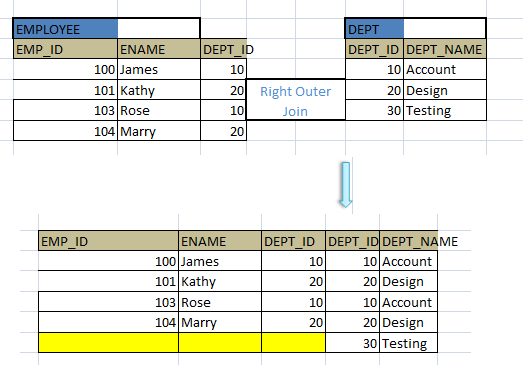Please note the difference I writing the query with left outer join and right outer join like the table and column positions, when used with ‘+’ operator then what is the position of operator etc.

## Full Outer Join

This is combination of both left and outer join. It displays all the matching columns from both the tables, and if it does not find any matching row, it displays NULL.

```SELECT d.DEPT_ID,
d.DEPT_NAME,
e.EMP_ID,
e.ENAME,
e.DEPT_ID
FROM EMP e FULL OUTER JOIN DEPT d
ON e.DEPT_ID = d.DEPT_ID;```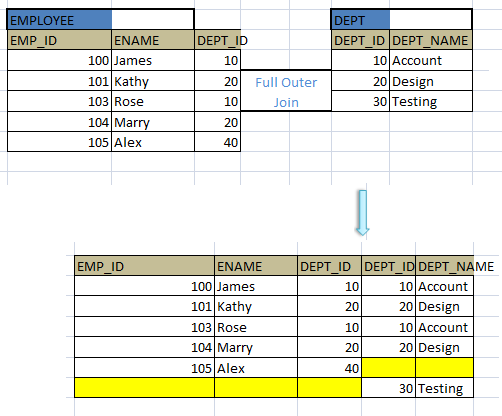Translate »